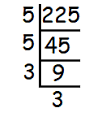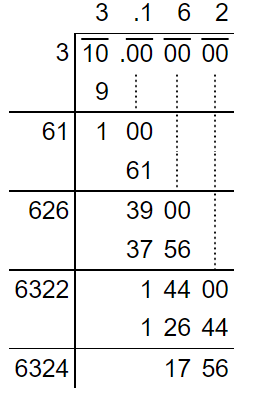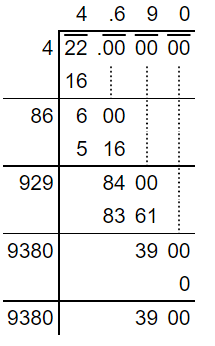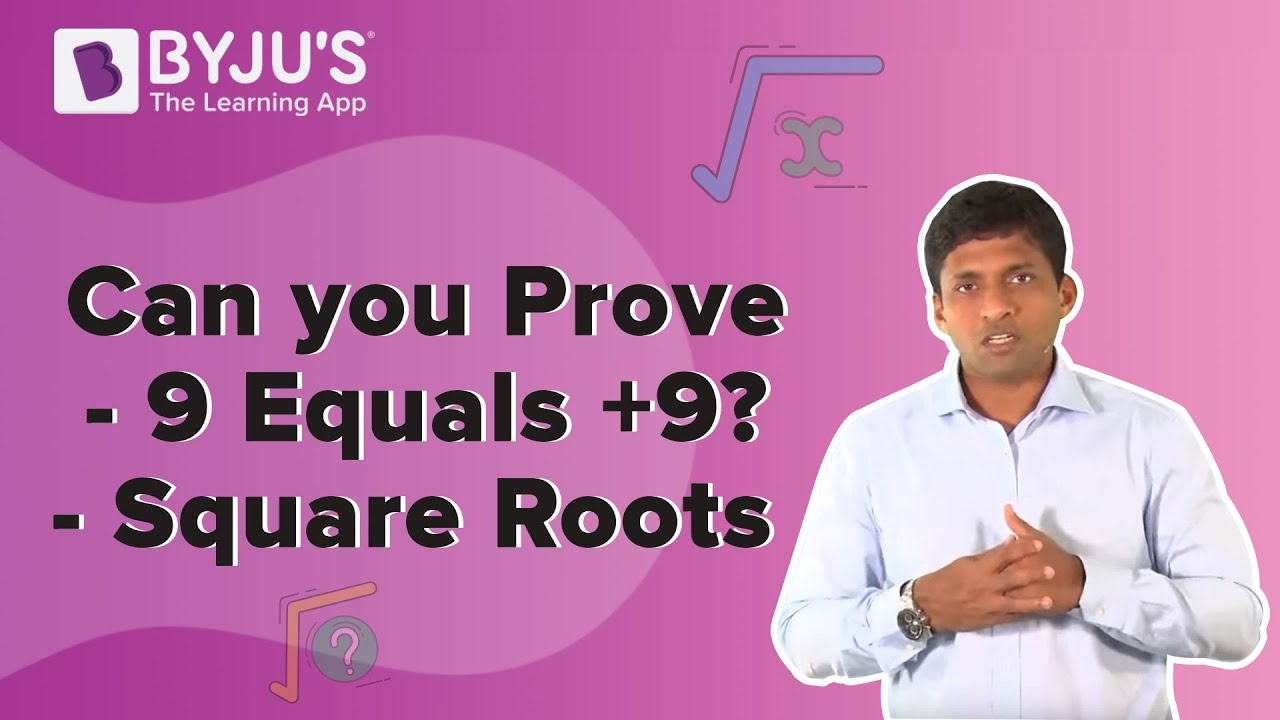Jet Set Go! All about Aeroplanes Jet Set Go! All about Aeroplanes

# Square Root Numbers

The square root numbers are the list of those numbers, which produce the original numbers when multiplied by themselves. The symbol used to represent the square root is a radical sign, i.e. ‘√’. The number present under the radical symbol is called ‘radicand’. This concept is one of the important concepts for Class 9 students.

To learn about square roots, we should first learn about squares of numbers. Then it will be easy for us to find the square roots of the numbers. When we multiply a number by itself, then it is said to be the square of that number. Suppose ‘a’ is a number and is multiplied by itself, then it is said to be squared. For example, 2 x 2 = 22 = 4. If we find the square root of 4, we get the original number, i.e. 2, since 4 is a perfect square. Therefore, it can be easily factored by the prime factorisation method to get the square root. See some more examples below:

• 3 squared = 32 = 9 & √9 = 3
• 7 squared = 72 = 49 & √49 = 7
• 11 squared = 112 = 121 & √121 = 11
• 12 squared = 122 = 144 & √144 = 12

## How To Find a Square Root of Numbers?

There are different methods to find the square root of given numbers. They are explained below along with an example in each case.

Method 1: Repeated Subtraction Method

The easiest and a simple method to find the square root of a given number is repeated subtraction. In this method, we need to subtract the consecutive odd numbers till we get 0. However, this method is suitable for only perfect square numbers, such as 4, 9, 16, 25, etc. Let us find the square root of 81 using this method.

81 – 1 = 80

80 – 3 = 77

77 – 5 = 72

72 – 7 = 65

65 – 9 = 56

56 – 11 = 45

45 – 13 = 32

32 – 15 = 17

17 – 17 = 0

Here, we subtracted 9 consecutive odd numbers. So, the square root of 81 is 9.

Method 2: Prime Factorisation Method

In this method, we need to express the given number as the product of its prime factors. This method is also applicable for perfect squares. Let’s look at the example given below to understand how to find the square root of a number using prime factorisation.

Example:

Consider the number 225.The product of prime factors of 225 is:

225 = 3 × 3 × 5 × 5

Take the square root on both sides.

√225 = √(3 × 3 × 5 × 5)

= √(32 × 52)

= 3 × 5

= 15

Thus, the square root of 225 is 15.

Method 3: Long Division Method

Finding the square root of very large numbers or imperfect squares could be a difficult task. Hence, we use the long division method to find the square root of such numbers in an easy and fastest way.

Let us find the square root of 10 using this method.√10 = 3.162…

Therefore, the square root of 10 upto 3 decimal places is 3.162.

## Square Root Numbers List

Here is the list of the square of numbers and their square roots. The table given below can be memorised by students easily so that they can solve questions based on these square root values.

 Number (n) Square (n2) Square root (√n) 1 1 1 2 4 1.4142 3 9 1.7321 4 16 2 5 25 2.2361 6 36 2.4495 7 49 2.6458 8 64 2.8284 9 81 3 10 100 3.1623 11 121 3.3166 12 144 3.4641 13 169 3.6056 14 196 3.7417 15 225 3.8730 16 256 4 17 289 4.1231 18 324 4.2426 19 361 4.3589 20 400 4.4721 21 441 4.5826 22 484 4.6904 23 529 4.7958 24 576 4.8990 25 625 5 26 676 5.0990 27 729 5.1962 28 784 5.2915 29 841 5.3852 30 900 5.4772 31 961 5.5678 32 1024 5.6569 33 1089 5.7446 34 1156 5.8310 35 1225 5.9161 36 1296 6 37 1369 6.0828 38 1444 6.1644 39 1521 6.2450 40 1600 6.3246 41 1681 6.4031 42 1764 6.4807 43 1849 6.5574 44 1936 6.6332 45 2025 6.7082 46 2116 6.7823 47 2209 6.8557 48 2304 6.9282 49 2401 7 50 2500 7.0711

### Solved Examples

Question 1:

Find the square root of 169 using the repeated subtraction method.

Solution:

Given number: 169

Let us subtract the consecutive odd numbers from 169 as shown below:

169 – 1 = 168

168 – 3 = 165

165 – 5 = 160

160 – 7 = 153

153 – 9 = 144

144 – 11 = 133

133 – 13 = 120

120 – 15 = 105

105 – 17 = 88

88 – 19 = 69

69 – 21 = 48

48 – 23 = 25

25 – 25 = 0

Here, 13 consecutive odd numbers are subtracted.

Therefore, the square root of 169 is 13.

Question 2:

Find the square root of 22 using a suitable method.

Solution:

As 22 is not a perfect square number, we should apply the long division method.

###√22 = 4.690…

Hence, the square root of 22 upto 3 decimal places is 4.690.

### Square Root Problems

Based on the table above we can solve the questions given here.

1. Solve √50 + √2 + √9
2. Multiply: √3 and √7
3. Divide: √49 by 7
4. Simplify: (√8 x √9) + √89
5. Simplify: 2√4+3√9+5√25

### Video LessonTest your knowledge on Square Root Numbers

#### 1 Comment

1. Guhiram Bhumij

Nice teaching I am impressed